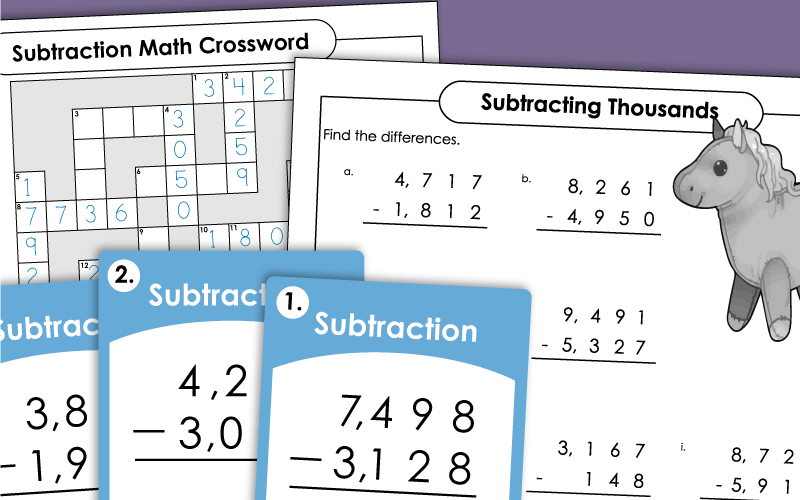# Subtraction: 4-Digit Numbers

This page is filled with printable worksheets for teaching and reviewing subtraction of four-digit numbers. In addition to traditional worksheets, you'll find task cards, a math crossword puzzle, and cut-and-glue activities.## 4-Digit SubtractionWorksheets

Solve the four-digit subtraction problems to find the differences. (example 46,397 - 2,976)
Solve the subtraction problems to find the differences.  Requires subtraction across zero with 4-digit numbers. (example 4,000 - 1,374)
Here's another 4-digit subtraction worksheet, similar to the one above. This printable has ten vertical problems and two word problems.
Find the differences to the subtraction problems to complete the math crossword.
Subtract numbers in the shapes shown. For instance: Subtract the number in the hexagon from the number in the pentagon.
Cut apart the math cards and solve the 4-digit subtraction problems. Then sort the differences into two categories on the t-chart: "Even Differences" and "Odd Differences."
This worksheet has pictures of subtraction problems that somebody has already tried to solve. But there are mistakes in their work. Find the errors and explain where they went wrong.
First use the cypher key to decode the mystery symbols into regular numbers. Then subtract.
(example: 6,526 - 2,818)
On this activity, students will decode the picture symbols into 4-digit numbers. Then they'll subtract.
Use the grid puzzle to solve the subtraction problems; 4-digit subtraction with zeros. (example 3,400 - 454)
Solve the 4-digit minus 3-digit subtraction problems. Glue the answers on the grid to see an astronaut picture.
Mixture of different types of subtraction problems, including a couple of word problems.  (3 and 4-digit)

## QR Code Subtraction

Scan the QR codes on this worksheet with a smartphone or iPad to view subtraction word problems. Solve and show your work in the space provided.
(Note: This worksheet requires students to use a smartphone or tablet with a QR code scanner.)
This is a set of 30 subtraction task cards. First students solve the 4-digit subtraction problems. Then they scan the QR codes with an iPad or smartphone to check their answers.
(Note: This worksheet requires students to use a smartphone or tablet with a QR code scanner.)

These thirty task cards have 4-digit subtraction problems on them. You can laminate the cards, and have students solve the problems with a dry-erase marker. Or use them to build a learning center. Or post a card each morning for your students to solve.
Use these task cards for learning centers, math lessons, math games, or review work. Most subtraction problems require borrowing across zero. Includes 30 task cards and an answer key.

## Mental Math(Subtraction Patterns)

Why couldn't the music teacher get into her classroom? To learn the answer to the funny riddle, kids must solve a series of mental math problems. All problems have 3, 4, or 5-digit numbers. (example: 9,000-4,000)

What did the duck eat for lunch? Solve the 4-digit addition and subtraction problems and match the letters with the answers to find out.
This page contains part-part-whole diagrams (also known as tape diagrams or bar models). Students subtract or add to find the missing number. Then they write an addition and subtraction fact illustrated by the model.
Here is another tape diagram (bar model) worksheet with seven problems. This version has empty space for students to show their work on the right-hand side.
Add and subtract 5-digit numbers. Find the answer to all problems to solve the riddle: What should you do when you see a blue elephant?
Complete seven bar models and write number sentences for each. This page requires students to demonstrate 5-digit addition and subtraction.
Complete the bar models by adding or subtracting 5-digit numbers. Then write an addition and subtraction fact to go along with each diagram.

## Subtraction WorksheetGenerator

Subtraction Worksheet Generator

Use this online tool to generate your own, customized subtraction worksheets with any number of digits.

3-Digit Subtraction

More Subtraction

Here you'll find links to all of our subtraction worksheet pages. This includes subtraction with and without regrouping, decimal subtraction, and subtracting fractions.

Math Worksheets

S.T.W. has thousands of math worksheets, covering a wide variety of topics, including operations, fractions, geometry, money, time, algebra, graphing, probability, and measurement.

My Account
Site Information